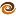EmptyCrate

About Me | Contact Me | Training |ChaiScript.com | YouTube Channel

Chapter 4 of Real World Haskellbegins with some details about functions with side effects and interacting with the outside world. Normally, a Haskell function is pure and may not have any side effects outside of itself. However, it is necessary to allow for side effects for reading and writing files, including standard input and output. The “do” keyword is used when we may have interactions with the outside world.

Infix Functions

Haskell allows you to define and use any function as an infix function.

a `plus` b = a + b

The infix notation is purely a convenience, or syntactic sugar as we might say, so the function can be called either by infix or prefix notation.

3 `plus` 2
plus 3 2

For me the added necessity of using back-ticks around the function name makes me wonder about the usefulness of this syntax. Although, the examples given in the book such as:

"end" `isSuffixOf` "the end"

Force me to admit that it can aid in code readability. Working With Lists The next section deals with all manner of list functions and working with lists which I will not catalogue here. The thing that did interest me in this section was this little tidbit from the authors:

Haskell’s type system makes it an interesting challenge to write functions that take variable numbers of arguments. So if we want to zip three lists together, we call zip3 or zipWith3, and so on up to zip7 and zipWith7.

It seems as though Haskell is dealing with the same kind of problems that variadic templates are meant to address. The main difference is that even with the current version of C++ you could choose to create 6 functions that take 2-7 arguments and name them all “zip” so you get the utility of having one function name and letting the compiler sort out for you which version to use.

Tail Recursion

The book goes on to discuss the fact that normal looping constructs do not exist in Haskell and recursion, particularly tail recursion - for performance reasons - is to be used. The book presents the following example for a tail recursive summation example:

-- file: ch04/Sum.hs
mySum xs = helper 0 xs
where helper acc (x:xs) = helper (acc + x) xs
helper acc _      = acc`

This is one point where the book loses me. The following version of a summation function makes a lot more sense to me. It is shorter, more readable, does not need an accumulator variable and is still tail recursive. I would very much like for a Haskell expert to be explain to me why one version would be preferable to the other. ` mySum (x:xs) = x + mySum(xs) mySum [] = 0`

Reading further we learn that the pattern used above, with an accumulator, is to prepare us for the use of the foldl and foldr functions, which are designed with just this pattern in mind

Space Leaks

Because of the lazy evaluation aspects of Haskell it is possible for functions such as “foldl” to consume far more memory then they should as the recursive call stack is generated and stored for large expressions. Instead, the function foldl’ (foldl-prime) should be used, which does not perform lazy evaluation.

Lambda Functions

Lambdas - anonymous functions - are introduced with a \ character. The best illustration for me of the way lambdas can be used is with this simple example of the integer parser from earlier in this chapter:

asInt_fold2 string = foldl (\acc x -> acc * 10 + digitToInt x) 0 string

In the above we create an unnamed function with two parameters, “acc” and “x”, which performs the work of parsing each digit of the passed in string of numbers. Lamdas are limited to only one clause, however, so they cannot do full pattern matching like named functions.

Partial Function Application

Partial function application simply means that if you leave off one or more parameters from a function call, a new function is generated and the parameters that you supplied are fixed permanently for the new function that is generated. We can keep building on our “asInt” function to show this example as well:

--The process of fixing a parameter is also known as "currying"
asInt_curry = foldl (\acc x -> acc * 10 + digitToInt x) 0

In this example we have just left off the only parameter (“string”) from the previous version because it was the last parameter that we were passing to foldl. In this way, a new function has been generated from our call to foldl that takes one parameter. My explanation seems weak, but if you can read the example, you get the idea.

seq

The seq function is used to force the evaluation of a variable, essentially short circuiting the lazy-evaluation feature of Haskell. It is useful for reducing space leaks in your code.

My Videos

Related